### IMO Shortlist 2012 problem C5

Kvaliteta:
Avg: 0,0
Težina:
Avg: 8,0
The columns and the row of a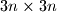$3n \times 3n$ square board are numbered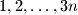$1,2,\ldots ,3n$. Every square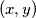$(x,y)$ with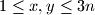$1 \leq x,y \leq 3n$ is colored asparagus, byzantium or citrine according as the modulo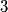$3$ remainder of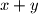$x+y$ is$0,1$ or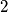$2$ respectively. One token colored asparagus, byzantium or citrine is placed on each square, so that there are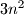$3n^2$ tokens of each color.
Suppose that on can permute the tokens so that each token is moved to a distance of at most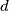$d$ from its original position, each asparagus token replaces a byzantium token, each byzantium token replaces a citirine token, and each citrine token replaces an aspargus token. Prove that it is possible to permute the tokens so that each token is moved to a distance of at most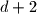$d+2$ from its original position, and each square contains a token with the same color as the square.
Izvor: Međunarodna matematička olimpijada, shortlist 2012